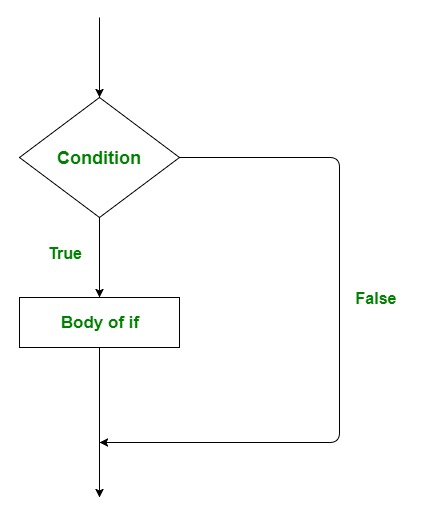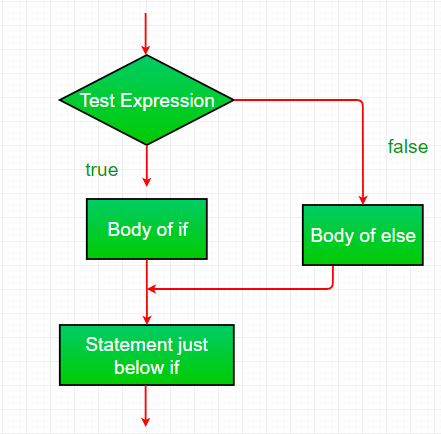GFG App
Open AppBrowser
Continue

The if-statement in Programming Language alone tells us that if a condition is true it will execute a block of statements and if the condition is false it won’t. But what if we want to do something else if the condition is false? Here comes the R Programming else statement. We can use the else statement with the if statement to execute a block of code when the condition is false.

### Syntax of if-else statement in R Language

```if (condition) {
# code to be executed if condition is TRUE
} else {
# code to be executed if condition is FALSE
}```if-else statement in R

## Working of if-else statements in R Programming

• Control falls into the if block.
• The flow jumps to Condition.
• Condition is tested.
• If the Condition yields true, goto Step 4.
• If the Condition yields false, goto Step 5.
• The if-block or the body inside the if is executed.
• The else block or the body inside the else is executed.
• Flow exits the if-else block.

### Flowchart if-else statement in RR – if-else statement

Example

## R

 `x <- 5` ` `  `# Check value is less than or greater than 10 ` `if``(x > 10)` `{ ` `    ``print``(``paste``(x, ``"is greater than 10"``)) ` `} ``else` `{ ` `    ``print``(``paste``(x, ``"is less than 10"``)) ` `}`

Output

` "5 is less than 10"`

Here in the above code, Firstly, x is initialized to 5, then the if-condition is checked(x > 10), and it yields false. Flow enters the else block and prints the statement “5 is less than 10”.

Example

## R

 `x <- 5`   `# Check if value is equal to 10 ` `if``(x == 10)` `{ ` `    ``print``(``paste``(x, ``"is equal to 10"``)) ` `} ``else` `{ ` `    ``print``(``paste``(x, ``"is not equal to 10"``)) ` `}`

Output

` "5 is not equal to 10" `

## Nested if-else statement in R

The if-else statements in R can be nested together to form a group of statements and evaluate expressions based on the conditions one by one, beginning from the outer condition to the inner one by one respectively. An if-else statement within another if-else statement in R better justifies the definition.

### Syntax

```if(condition1){
# execute only if condition 1 satisfies
if(condition 2){
# execute if both condition 1 and 2 satisfy
}
}else{
}```

Example

## R

 `# define a variable` `x <- 15`   `# check the value of x using nested if-else statements` `if ``(x < 10) {` `  ``# if x is less than 10` `  ``print``(``"x is less than 10"``)` `} ``else` `{` `  ``# if x is greater than or equal to 10` `  ``if ``(x < 20) {` `    ``# if x is less than 20` `    ``print``(``"x is between 10 and 20"``)` `  ``} ``else` `{` `    ``# if x is greater than or equal to 20` `    ``print``(``"x is greater than or equal to 20"``)` `  ``}` `}`

Output

` "x is between 10 and 20"`
• In this example, we first define a variable x with the value of 15.
• Then we use a nested if-else statement to check the value of x.
• The outer if-else statement checks if x is less than 10. If it is, we print the message “x is less than 10”. If x is not less than 10, we move on to the nested if-else statement inside the else block.
• This nested if-else statement checks if x is less than 20. If it is, we print the message “x is between 10 and 20”. If x is not less than 20, we print the message “x is greater than or equal to 20”. Since x is 15, the code will print “x is between 10 and 20”.

Example

## R

 `# define variables for grades and income` `grades <- 85` `income <- 25000`   `# check eligibility for scholarship using nested if-else statements` `if ``(grades >= 80) {` `  ``# if grades are 80 or above` `  ``if ``(income <= 30000) {` `    ``# if income is 30,000 or less` `    ``print``(``"Congratulations, you are eligible for a scholarship!"``)` `  ``} ``else` `{` `    ``# if income is more than 30,000` `    ``print``(``"Sorry, your income is too high to qualify for a scholarship."``)` `  ``}` `} ``else` `{` `  ``# if grades are below 80` `  ``print``(``"Sorry, your grades are too low to qualify for a scholarship."``)` `}`

Output

` "Congratulations, you are eligible for a scholarship!"`
• In this example, we first define two variables, grades and income, to represent a student’s grades and income, respectively.
• Then we use a nested if-else statement to check if the student is eligible for a scholarship.
• The outer if-else statement checks if the student’s grades are 80 or above. If they are, we move on to the nested if-else statement inside the if block. This nested if-else statement checks if the student’s income is 30,000 or less.
•  If it is, we print the message “Congratulations, you are eligible for a scholarship!” If the student’s income is more than 30,000, we print the message “Sorry, your income is too high to qualify for a scholarship.” If the student’s grades are below 80, we print the message “Sorry, your grades are too low to qualify for a scholarship.”
•  This nested if-else statement can be expanded upon to include additional conditions, such as residency or enrollment status, to determine scholarship eligibility.

My Personal Notes arrow_drop_up